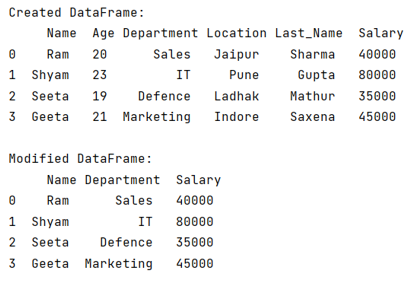# What is the fast way to drop columns in pandas DataFrame?

Given a Pandas DataFrame, we have to drop columns in it. By Pranit Sharma Last updated : September 23, 2023

Pandas is a special tool that allows us to perform complex manipulations of data effectively and efficiently. Inside pandas, we mostly deal with a dataset in the form of DataFrame. DataFrames are 2-dimensional data structures in pandas. DataFrames consist of rows, columns, and the data. The Data inside the DataFrame can be of any type.

## Problem statement

Given a Pandas DataFrame, we have to drop columns in it.

## Fast way to drop columns in pandas DataFrame

When we need to drop a specific column in pandas DataFrame, we use pandas.DataFrame.drop() method but here we need to drop multiple columns quickly, hence, we will first encapsulate all the columns in a list which we want to drop and then we will pass this list as a parameter inside pandas.DataFrame.drop() method.

### Syntax

```DataFrame.drop(
labels=None,
axis=0,
index=None,
columns=None,
level=None,
inplace=False,
errors='raise'
)
```
Note

To work with pandas, we need to import pandas package first, below is the syntax:

```import pandas as pd
```

Let us understand with the help of an example,

## Python program to drop columns in pandas DataFrame

```# Importing pandas package
import pandas as pd

# Creating a dictionary
d = {
'Name':['Ram','Shyam','Seeta','Geeta'],
'Age':[20,23,19,21],
'Department':['Sales','IT','Defence','Marketing'],
'Last_Name':['Sharma','Gupta','Mathur','Saxena'],
'Salary':[40000,80000,35000,45000]
}

# Creating dataframe
df = pd.DataFrame(d)

# Display DataFrame
print("Created DataFrame:\n",df,"\n")

# Encapsulating all the columns in a list
# which we want to delete
col_to_be_deleted = ['Age','Location','Last_Name']

# Deleting all three columns quickly by using df.drop()
df.drop(col_to_be_deleted , axis=1 , inplace=True)

# Display Modified DataFrame
print("Modified DataFrame:\n",df)
```

### Output

The output of the above program is: